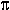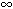DOC HOME SITE MAP MAN PAGES GNU INFO SEARCH PRINT BOOK

# trig(3M)

sin, cos, tan, asin, acos, atan, atan2 -- trigonometric functions

## Synopsis

```   cc [flag ...] file ...  -lm [library ...]

#include <math.h>

double sin(double x);

float sinf(float x);

long double sinl(long double x);

double cos(double x);

float cosf(float x);

long double cosl(long double x);

double tan(double x);

float tanf(float x);

long double tanl(long double x);

double asin(double x);

float asinf(float x);

long double asinl(long double x);

double acos(double x);

float acosf(float x);

long double acosl(long double x);

double atan(double x);

float atanf(float x);

long double atanl(long double x);

double atan2(double y, double x);

float atan2f(float y, float x);

long double atan2l(long double y, long double x);
```

## Description

sin, cos, tan, the single-precision versions sinf, cosf, tanf and the long double-precision versions sinl, cosl, and tanl return, respectively, the sine, cosine, and tangent of their argument, x, measured in radians.

asin, asinf and asinl return the arcsine of x, in the range [-/2,+/2].

acos, acosf and acosl return the arccosine of x, in the range [0,+].

atan, atanf and atanl return the arctangent of x, in the range (-/2,+/2).

atan2, atan2f and atan2l return the arctangent of y/x in the range (-,+], using the signs of both arguments to determine the quadrant of the return value.

### Errors

If the magnitude of the argument to asin, asinf, asinl, acos, acosf or acosl is greater than 1, they return IEEE NaN on systems that support it and raise the invalid operation exception. Otherwise, they return zero. In either case, errno is set to EDOM.

On systems that support signed zeroes, if the second argument to atan2, atan2f or atan2l is +0 and the first argument is ±0, these functions return ±0, depending on the sign of the first argument. When the second argument to atan2, atan2f or atan2l is -0 and the first argument is ±0, these functions return ±, depending on the sign of the first argument. On systems that do not support signed zeroes, if both arguments to atan2, atan2f or atan2l are zero, the return value is zero. In each of these cases, errno is set to EDOM.

On systems that support IEEE infinity, if the argument to sin, sinf, sinl, cos, cosf, cosl, tan, tanf or tanl is ±, they return IEEE NaN, raise the invalid operation exception and set errno to EDOM.

On systems that support IEEE NaN, if any of the arguments to any of these functions is a quiet NaN, that value is returned. If any of the arguments is a signaling NaN, a quiet NaN is returned and the invalid operation exception is raised. In either case, errno is set to EDOM.

If the program was compiled with the -Xt compilation mode, if the magnitude of the argument to asin, asinf, asinl, acos, acosf or acosl is greater than 1 or when both arguments to atan2, atan2f or atan2l are zero, they return zero and set errno to EDOM. In addition, a message indicating DOMAIN error is printed on the standard error output. These error handling procedures may be changed with the function matherr.

## References

cc(1), matherr(3M)